# 【Unity3D开发小游戏】炸弹人游戏

@[toc]

## 二、资源下载

UI资源和源代码请搜索QQ群：1040082875下载

## 三、让我们开始吧

Unity 5.0.1f1

### 2.创建级别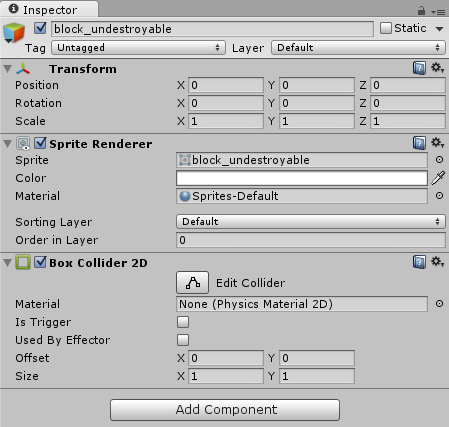现在，街区是物理世界的一部分，就像一堵墙。

### 3.炸弹人

• 向左走
• 向右走
• 向上走
• 向下走
• Idle停滞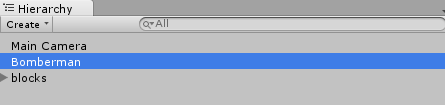我们需要一个动画控制器，但是我们不需要其他的控制器，让我们来删除其他4个控制器：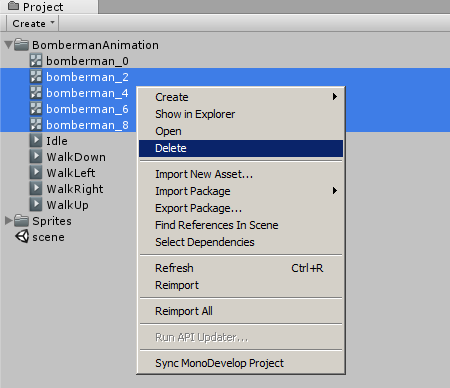现在我们有5个动画，然后还有一个动画控制器：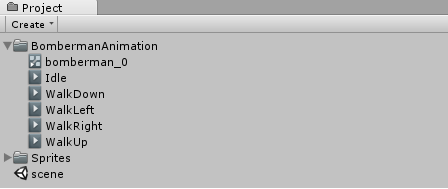动画控制器参数 让我们双击bomberman_0控制器，然后我们就可以进入Animator：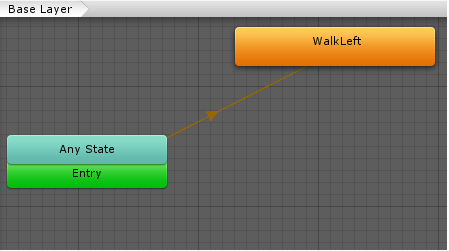这里我们看到的是控制炸弹人的动画状态机，它会自动在不同的动画之间切换，取决于我们给它传递的参数。

• Y>0意味着WalkUp向上
• Y<0意味着WalkDown向下
• X>0意味着WalkRight向左
• X<0意味着WalkLeft向右
• X=0和Y=0意味着Idle不动

• WalkUp向上Condition设置为Y>0
• WalkDown向下Condition设置为Y<0
• WalkRight向左Condition设置为X>0
• WalkLeft向右Condition设置为X<0
• Idle待机Condition设置为X=0和Y=0

### 5.炸弹人移动

``````using UnityEngine;
using System.Collections;

public class Move : MonoBehaviour {

void FixedUpdate () {

}
}
``````

``````void FixedUpdate () {
// Check Input Axes
float h = Input.GetAxisRaw("Horizontal");
float v = Input.GetAxisRaw("Vertical");
}
``````

``````using UnityEngine;
using System.Collections;

public class Move : MonoBehaviour {
public float speed = 6;

void FixedUpdate () {
// Check Input Axes
float h = Input.GetAxisRaw("Horizontal");
float v = Input.GetAxisRaw("Vertical");
}
}
``````

``````void FixedUpdate () {
// Check Input Axes, then use velocity
float h = Input.GetAxisRaw("Horizontal");
float v = Input.GetAxisRaw("Vertical");
GetComponent<Rigidbody2D>().velocity = new Vector2(h, v) * speed;
}
``````

``````void FixedUpdate () {
// Check Input Axes, then use velocity
float h = Input.GetAxisRaw("Horizontal");
float v = Input.GetAxisRaw("Vertical");
GetComponent<Rigidbody2D>().velocity = new Vector2(h, v) * speed;

// Set Animation Parameters
GetComponent<Animator>().SetInteger("X", (int)h);
GetComponent<Animator>().SetInteger("Y", (int)v);
}
``````

### 6.炸弹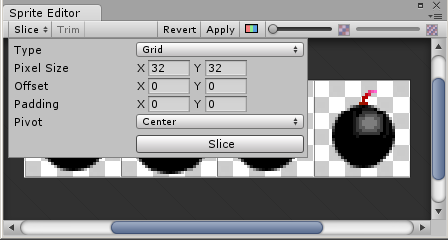炸弹动画 将它们拖到场景中：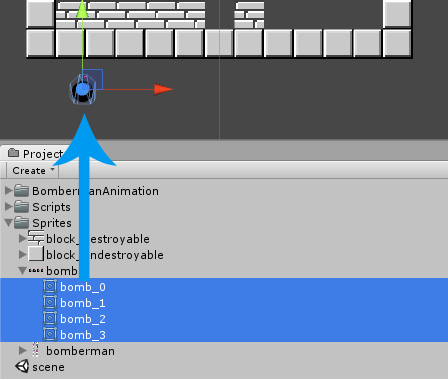然后将动画文件保存到Assets/BombAnimation文件夹中，命名为Bomb.anim

``````using UnityEngine;
using System.Collections;

public class BombDrop : MonoBehaviour {

// Update is called once per frame
void Update () {

}
}
``````

``````using UnityEngine;
using System.Collections;

public class BombDrop : MonoBehaviour {
public GameObject bombPrefab;

void Update () {
if (Input.GetKeyDown(KeyCode.Space)) {
Vector2 pos = transform.position;
pos.x = Mathf.Round(pos.x);
pos.y = Mathf.Round(pos.y);
Instantiate(bombPrefab, pos, Quaternion.identity);
}
}
}
``````

``````using UnityEngine;
using System.Collections;

public class DestroyAfter : MonoBehaviour {

// Use this for initialization
void Start () {

}
}
``````

``````using UnityEngine;
using System.Collections;

public class DestroyAfter : MonoBehaviour {
public float time = 3;

// Use this for initialization
void Start () {
Destroy(gameObject, time);
}
}
``````

``````using UnityEngine;
using System.Collections;

public class Bomb : MonoBehaviour {

void OnDestroy() {

}
}
``````

``````using UnityEngine;
using System.Collections;

public class Bomb : MonoBehaviour {
// Explosion Prefab
public GameObject explosionPrefab;

void OnDestroy() {
Instantiate(explosionPrefab, transform.position, Quaternion.identity);
}
}
``````

### 7.爆炸效果

``````using UnityEngine;
using System.Collections;

public class Explosion : MonoBehaviour {

void OnTriggerEnter2D(Collider2D co) {
// Do Stuff...
}
}
``````

``````using UnityEngine;
using System.Collections;

public class Explosion : MonoBehaviour {

void OnTriggerEnter2D(Collider2D co) {
if (!co.gameObject.isStatic)
Destroy(co.gameObject);
}
}
``````

### 8.制造怪物

• worm_0, 1, 2 左
• worm_3, 4, 5 右
• worm_6, 7, 8 下
• worm_9, 10, 11 上
• worm_12, 13 静止

### 10.怪物移动

``````using UnityEngine;
using System.Collections;

public class Worm : MonoBehaviour {

// Use this for initialization
void Start () {

}
}
``````

``````using UnityEngine;
using System.Collections;

public class Worm : MonoBehaviour {
public float speed = 2;

// Use this for initialization
void Start () {

}
}
``````

``````Vector2 randDir() {
// Set one component to -1/0/1 and one to 0
int r = Random.Range(-1, 2);
return (Random.value < 0.5) ? new Vector2(r, 0) : new Vector2(0, r);
}
``````

``````bool isValidDir(Vector2 dir) {
// Linecast to find out if anything is between worm and worm+dir
Vector2 pos = transform.position;
RaycastHit2D hit = Physics2D.Linecast(pos + dir, pos);
return (hit.collider.gameObject == gameObject);
}
``````

``````void changeDir() {
Vector2 dir = randDir();
if (isValidDir(dir)) {
GetComponent<Rigidbody2D>().velocity = dir * speed;
GetComponent<Animator>().SetInteger("X", (int)dir.x);
GetComponent<Animator>().SetInteger("Y", (int)dir.y);
}
}
``````

``````// Use this for initialization
void Start () {
InvokeRepeating("changeDir", 0, 0.5f);
}
``````

### 12.摘要

• 只允许同时存在一定数量的炸弹
• 使炸弹人也收到炸弹的影响
• 使怪物易受炸弹影响
• 创建游戏菜单
• 为多人版本添加更多角色
• 把开关或者其他楼层放到积木后面